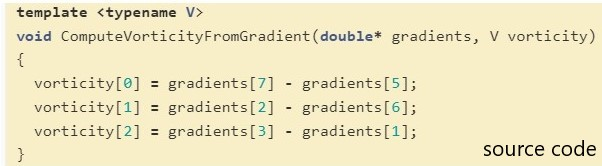I just started learning CFD
My problem is that the function of calculating vorticity in the filter is in the source code. I don’t understand the mathematical equation it represents.
Does anyone know how to write this source code as a mathematical equation.
The picture is the mathematical equation I want to calculate the vorticityHello,

The variable gradients represents the velocity gradient tensor, and each component of the gradients array is as follows: